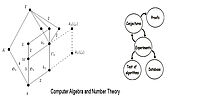Sie haben Javascript deaktiviert!
Sie haben versucht eine Funktion zu nutzen, die nur mit Javascript möglich ist. Um sämtliche Funktionalitäten unserer Internetseite zu nutzen, aktivieren Sie bitte Javascript in Ihrem Browser.# Exponents

Here we provide the explicit results of all imaginary multiquadratic number fields with exponent 1, 3, and 5. Furthermore we give links to the C and Julia programs we used.

The scientific content is given in the following two articles:

Imaginary quadratic number fields with small exponent

Imaginary biquadratic number fields with exponent 1,3,5.

Imaginary triquadratic number fields with exponent 1,3,5.

##### C-Programs

The following two c-programs can be used to compute all imaginary quadratic number fields with exponent less or equal to 8 and discriminant bound 3.1·1020.

No small split.c

List of fields

##### Julia Programs

The following file contains the Julia code. You need to install Julia including the Hecke and the Markdown package. The function M1 computes the imginary quadratic number fields of given exponent. The functions M2a, M2b, M3a, and M3b compute the corresponding families.